# Time Series Regression VIII: Lagged Variables and Estimator Bias

This example shows how lagged predictors affect least-squares estimation of multiple linear regression models. It is the eighth in a series of examples on time series regression, following the presentation in previous examples.

### Introduction

Many econometric models are dynamic, using lagged variables to incorporate feedback over time. By contrast, static time series models represent systems that respond exclusively to current events.

Lagged variables come in several types:

• Distributed Lag (DL) variables are lagged values ${x}_{t-k}$ of observed exogenous predictor variables ${x}_{t}$.

• Autoregressive (AR) variables are lagged values ${y}_{t-k}$ of observed endogenous response variables ${y}_{t}$.

• Moving Average (MA) variables are lagged values ${e}_{t-k}$ of unobserved stochastic innovations processes ${e}_{t}$.

Dynamic models are often constructed using linear combinations of different types of lagged variables, to create ARMA, ARDL, and other hybrids. The modeling goal, in each case, is to reflect important interactions among relevant economic factors, accurately and concisely.

Dynamic model specifications ask the question: Which lags are important? Some models, such as seasonal models, use lags at distinct periods in the data. Other models base their lag structure on theoretical considerations of how, and when, economic agents react to changing conditions. In general, lag structures identify the time delay of the response to known leading indicators.

However, lag structures must do more than just represent the available theory. Because dynamic specifications produce interactions among variables that can affect standard regression techniques, lag structures must also be designed with accurate model estimation in mind.

### Specification Issues

Consider the multiple linear regression (MLR) model:

${y}_{t}={Z}_{t}\beta +{e}_{t},$

where ${y}_{t}$ is an observed response, ${Z}_{t}$ includes columns for each potentially relevant predictor variable, including lagged variables, and ${e}_{t}$ is a stochastic innovations process. The accuracy of estimation of the coefficients in $\beta$ depends on the constituent columns of ${Z}_{t}$, as well as the joint distribution of ${e}_{t}$. Selecting predictors for ${Z}_{t}$ that are both statistically and economically significant usually involves cycles of estimation, residual analysis, and respecification.

Classical linear model (CLM) assumptions, discussed in the example Time Series Regression I: Linear Models, allow ordinary least squares (OLS) to produce estimates of $\beta$ with desirable properties: unbiased, consistent, and efficient relative to other estimators. Lagged predictors in ${Z}_{t}$, however, can introduce violations of CLM assumptions. Specific violations depend on the types of lagged variables in the model, but the presence of dynamic feedback mechanisms, in general, tends to exaggerate the problems associated with static specifications.

Model specification issues are usually discussed relative to a data-generating process (DGP) for the response variable ${y}_{t}$. Practically, however, the DGP is a theoretical construct, realized only in simulation. No model ever captures real-world dynamics entirely, and model coefficients in $\beta$ are always a subset of those in the true DGP. As a result, innovations in ${e}_{t}$ become a mix of the inherent stochasticity of the process and a potentially large number of omitted variables (OVs). Autocorrelations in ${e}_{t}$ are common in econometric models where OVs exhibit persistence over time. Rather than comparing a model to a theoretical DGP, it is more practical to evaluate whether, or to what degree, dynamics in the data have been distinguished from autocorrelations in the residuals.

Initially, lag structures may include observations of economic factors at multiple, proximate times. However, observations at time t are likely to be correlated with observations at times t - 1, t - 2, and so forth, through economic inertia. Thus, a lag structure may overspecify the dynamics of the response by including a sequence of lagged predictors with only marginal contributions to the DGP. The specification will overstate the effects of past history, and fail to impose relevant restrictions on the model. Extended lag structures also require extended presample data, reducing the sample size and decreasing the number of degrees of freedom in estimation procedures. Consequently, overspecified models may exhibit pronounced problems of collinearity and high estimator variance. The resulting estimates of $\beta$ have low precision, and it becomes difficult to separate individual effects.

To reduce predictor dependencies, lag structures can be restricted. If the restrictions are too severe, however, other problems of estimation arise. A restricted lag structure may underspecify the dynamics of the response by excluding predictors that are actually a significant part of the DGP. This leads to a model that underestimates the effects of past history, forcing significant predictors into the innovations process. If lagged predictors in ${e}_{t}$ are correlated with proximate lagged predictors in ${Z}_{t}$, the CLM assumption of strict exogeneity of the regressors is violated, and OLS estimates of $\beta$ become biased and inconsistent.

Specific issues are associated with different types of lagged predictors.

Lagged exogenous predictors ${x}_{t-k}$, by themselves, do not violate CLM assumptions. However, DL models are often described, at least initially, by a long sequence of potentially relevant lags, and so suffer from the problems of overspecification mentioned above. Common, if somewhat ad hoc, methods for imposing restrictions on the lag weights (that is, the coefficients in $\beta$) are discussed in the example Time Series Regression IX: Lag Order Selection. In principle, however, the analysis of a DL model parallels that of a static model. Estimation issues related to collinearity, influential observations, spurious regression, autocorrelated or heteroscedastic innovations, etc. must still be examined.

Lagged endogenous predictors ${y}_{t-k}$ are more problematic. AR models introduce violations of CLM assumptions that lead to biased OLS estimates of $\beta$. Absent any other CLM violations, the estimates are, nevertheless, consistent and relatively efficient. Consider a simple first-order autoregression of ${y}_{t}$ on ${y}_{t-1}$:

${y}_{t}=\beta {y}_{t-1}+{e}_{t}.$

In this model, ${y}_{t}$ is determined by both ${y}_{t-1}$ and ${e}_{t}$. Shifting the equation backwards one step at a time, ${y}_{t-1}$ is determined by both ${y}_{t-2}$ and ${e}_{t-1}$, ${y}_{t-2}$ is determined by both ${y}_{t-3}$ and ${e}_{t-2}$, and so forth. Transitively, the predictor ${y}_{t-1}$ is correlated with the entire previous history of the innovations process. Just as with underspecification, the CLM assumption of strict exogeneity is violated, and OLS estimates of $\beta$ become biased. Because $\beta$ must absorb the effects of each ${e}_{t-k}$, the model residuals no longer represent true innovations .

The problem is compounded when the innovations in an AR model are autocorrelated. As discussed in the example Time Series Regression VI: Residual Diagnostics, autocorrelated innovations in the absence of other CLM violations produce unbiased, if potentially high variance, OLS estimates of model coefficients. The major complication, in that case, is that the usual estimator for the standard errors of the coefficients becomes biased. (The effects of heteroscedastic innovations are similar, though typically less pronounced.) If, however, autocorrelated innovations are combined with violations of strict exogeneity, like those produced by AR terms, estimates of $\beta$ become both biased and inconsistent.

If lagged innovations ${e}_{t-k}$ are used as predictors, the nature of the estimation process is fundamentally changed, since the innovations cannot be directly observed. Estimation requires that the MA terms be inverted to form infinite AR representations, and then restricted to produce a model that can be estimated in practice. Since restrictions must be imposed during estimation, numerical optimization techniques other than OLS, such as maximum likelihood estimation (MLE), are required. Models with MA terms are considered in the example Time Series Regression IX: Lag Order Selection.

### Simulating Estimator Bias

To illustrate the estimator bias introduced by lagged endogenous predictors, consider the following DGP:

${y}_{t}={\beta }_{0}{y}_{t-1}+{e}_{t},$

${e}_{t}={\gamma }_{0}{e}_{t-1}+{\delta }_{t},$

${\delta }_{t}\sim N\left(0,{\sigma }^{2}\right).$

We run two sets of repeated Monte Carlo simulations of the model. The first set uses normally and independently distributed (NID) innovations with ${\gamma }_{0}=0$. The second set uses AR(1) innovations with $|{\gamma }_{0}|>0$ .

% Build the model components: beta0 = 0.9; % AR(1) parameter for y_t gamma0 = 0.2; % AR(1) parameter for e_t AR1 = arima('AR',beta0,'Constant',0,'Variance',1); AR2 = arima('AR',gamma0,'Constant',0,'Variance',1); % Simulation sample sizes: T = [10,50,100,500,1000]; numSizes = length(T); % Run the simulations: numObs = max(T); % Length of simulation paths numPaths = 1e4; % Number of simulation paths burnIn = 100; % Initial transient period, to be discarded sigma = 2.5; % Standard deviation of the innovations E0 = sigma*randn(burnIn+numObs,numPaths,2); % NID innovations E1Full = E0(:,:,1); Y1Full = filter(AR1,E1Full); % AR(1) process with NID innovations E2Full = filter(AR2,E0(:,:,2)); Y2Full = filter(AR1,E2Full); % AR(1) process with AR(1) innovations clear E0 % Extract simulation data, after transient period: Y1 = Y1Full(burnIn+1:end,:); % Y1(t) LY1 = Y1Full(burnIn:end-1,:); % Y1(t-1) Y2 = Y2Full(burnIn+1:end,:); % Y2(t) LY2 = Y2Full(burnIn:end-1,:); % Y2(t-1) clear Y1Full Y2Full % Compute OLS estimates of beta0: BetaHat1 = zeros(numSizes,numPaths); BetaHat2 = zeros(numSizes,numPaths); for i = 1:numSizes n = T(i); for j = 1:numPaths BetaHat1(i,j) = LY1(1:n,j)\Y1(1:n,j); BetaHat2(i,j) = LY2(1:n,j)\Y2(1:n,j); end end % Set plot domains: w1 = std(BetaHat1(:)); x1 = (beta0-w1):(w1/1e2):(beta0+w1); w2 = std(BetaHat2(:)); x2 = (beta0-w2):(w2/1e2):(beta0+w2); % Create figures and plot handles: hFig1 = figure; hold on hPlots1 = zeros(numSizes,1); hFig2 = figure; hold on hPlots2 = zeros(numSizes,1); % Plot estimator distributions: colors = winter(numSizes); for i = 1:numSizes c = colors(i,:); figure(hFig1); f1 = ksdensity(BetaHat1(i,:),x1); hPlots1(i) = plot(x1,f1,'Color',c,'LineWidth',2); figure(hFig2); f2 = ksdensity(BetaHat2(i,:),x2); hPlots2(i) = plot(x2,f2,'Color',c,'LineWidth',2); end % Annotate plots: figure(hFig1) hBeta1 = line([beta0 beta0],[0 (1.1)*max(f1)],'Color','c','LineWidth',2); xlabel('Estimate') ylabel('Density') title(['{\bf OLS Estimates of \beta_0 = ',num2str(beta0,2),', NID Innovations}']) legend([hPlots1;hBeta1],[strcat({'T = '},num2str(T','%-d'));['\beta_0 = ',num2str(beta0,2)]]) axis tight grid on hold off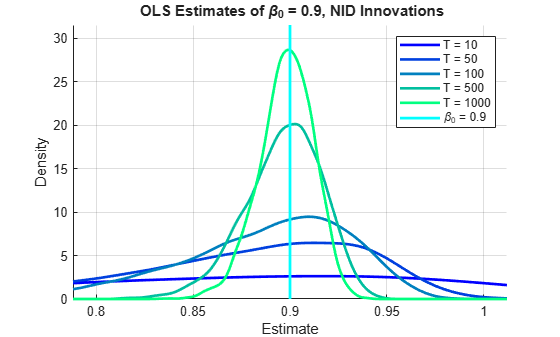figure(hFig2) hBeta2 = line([beta0 beta0],[0 (1.1)*max(f2)],'Color','c','LineWidth',2); xlabel('Estimate') ylabel('Density') title(['{\bf OLS Estimates of \beta_0 = ',num2str(beta0,2),', AR(1) Innovations}']) legend([hPlots2;hBeta2],[strcat({'T = '},num2str(T','%-d'));['\beta_0 = ',num2str(beta0,2)]]) axis tight grid on hold offIn all of the simulations above, ${\beta }_{0}=0.9$. The plots are the distributions of $\underset{}{\overset{ˆ}{{\beta }_{0}}}$ across multiple simulations of each process, showing the bias and variance of the OLS estimator as a function of sample size.

The skew of the distributions makes it difficult to visually assess their centers. Bias is defined as $E\left[\underset{}{\overset{ˆ}{{\beta }_{0}}}\right]-{\beta }_{0}$, so we use the mean to measure the aggregate estimate. In the case of NID innovations, a relatively small negative bias disappears asymptotically as the aggregate estimates increase monotonically toward ${\beta }_{0}$:

AggBetaHat1 = mean(BetaHat1,2); fprintf('%-6s%-6s\n','Size','Mean1')
Size Mean1 
for i = 1:numSizes fprintf('%-6u%-6.4f\n',T(i),AggBetaHat1(i)) end
10 0.7974 50 0.8683 100 0.8833 500 0.8964 1000 0.8981 

In the case of AR(1) innovations, aggregate estimates with a negative bias in small samples increase monotonically toward ${\beta }_{0}$, as above, but then pass through the DGP value at moderate sample sizes, and become progressively more positively biased in large samples:

AggBetaHat2 = mean(BetaHat2,2); fprintf('%-6s%-6s\n','Size','Mean2')
Size Mean2 
for i = 1:numSizes fprintf('%-6u%-6.4f\n',T(i),AggBetaHat2(i)) end
10 0.8545 50 0.9094 100 0.9201 500 0.9299 1000 0.9310 

The inconsistency of the OLS estimator in the presence of autocorrelated innovations is widely known among econometricians. That it nevertheless gives accurate estimates for a range of sample sizes has practical consequences that are less widely appreciated. We describe this behavior further in the section Dynamic and Correlation Effects.

The principal difference between the two sets of simulations above, in terms of OLS estimation, is whether or not there is a delay in the interaction between the innovations and the predictor. In the AR(1) process with NID innovations, the predictor ${y}_{t-1}$ is contemporaneously uncorrelated with ${e}_{t}$, but correlated with all of the previous innovations, as described earlier. In the AR(1) process with AR(1) innovations, the predictor ${y}_{t-1}$ becomes correlated with ${e}_{t}$ as well, through the autocorrelation between ${e}_{t}$ and ${e}_{t-1}$.

To see these relationships, we compute the correlation coefficients between ${y}_{t-1}$ and both ${e}_{t}$ and ${e}_{t-1}$, respectively, for each process:

% Extract innovations data, after transient period: E1 = E1Full(burnIn+1:end,:); % E1(t) LE1 = E1Full(burnIn:end-1,:); % E1(t-1) E2 = E2Full(burnIn+1:end,:); % E2(t) LE2 = E2Full(burnIn:end-1,:); % E2(t-1) clear E1Full E2Full % Preallocate for correlation coefficients: CorrE1 = zeros(numSizes,numPaths); CorrLE1 = zeros(numSizes,numPaths); CorrE2 = zeros(numSizes,numPaths); CorrLE2 = zeros(numSizes,numPaths); % Compute correlation coefficients: for i = 1:numSizes n = T(i); for j = 1:numPaths % With NID innovations: CorrE1(i,j) = corr(LY1(1:n,j),E1(1:n,j)); CorrLE1(i,j) = corr(LY1(1:n,j),LE1(1:n,j)); % With AR(1) innovations CorrE2(i,j) = corr(LY2(1:n,j),E2(1:n,j)); CorrLE2(i,j) = corr(LY2(1:n,j),LE2(1:n,j)); end end % Set plot domains: sigmaE1 = std(CorrE1(:)); muE1 = mean(CorrE1(:)); xE1 = (muE1-sigmaE1):(sigmaE1/1e2):(muE1+sigmaE1); sigmaLE1 = std(CorrLE1(:)); muLE1 = mean(CorrLE1(:)); xLE1 = (muLE1-sigmaLE1/2):(sigmaLE1/1e3):muLE1; sigmaE2 = std(CorrE2(:)); muE2 = mean(CorrE2(:)); xE2 = (muE2-sigmaE2):(sigmaE2/1e2):(muE2+sigmaE2); sigmaLE2 = std(CorrLE2(:)); muLE2 = mean(CorrLE2(:)); xLE2 = (muLE2-sigmaLE2):(sigmaLE2/1e2):(muLE2+sigmaLE2); % Create figures and plot handles: hFigE1 = figure; hold on hPlotsE1 = zeros(numSizes,1); hFigLE1 = figure; hold on hPlotsLE1 = zeros(numSizes,1); hFigE2 = figure; hold on hPlotsE2 = zeros(numSizes,1); hFigLE2 = figure; hold on hPlotsLE2 = zeros(numSizes,1); % Plot correlation coefficient distributions: colors = copper(numSizes); for i = 1:numSizes c = colors(i,:); figure(hFigE1) fE1 = ksdensity(CorrE1(i,:),xE1); hPlotsE1(i) = plot(xE1,fE1,'Color',c,'LineWidth',2); figure(hFigLE1) fLE1 = ksdensity(CorrLE1(i,:),xLE1); hPlotsLE1(i) = plot(xLE1,fLE1,'Color',c,'LineWidth',2); figure(hFigE2) fE2 = ksdensity(CorrE2(i,:),xE2); hPlotsE2(i) = plot(xE2,fE2,'Color',c,'LineWidth',2); figure(hFigLE2) fLE2 = ksdensity(CorrLE2(i,:),xLE2); hPlotsLE2(i) = plot(xLE2,fLE2,'Color',c,'LineWidth',2); end clear CorrE1 CorrLE1 CorrE2 CorrLE2 % Annotate plots: figure(hFigE1) xlabel('Correlation Coefficient') ylabel('Density') title('{\bf Sample Correlation of {\it y_{t-1}} and NID {\it e_t}}') legend(hPlotsE1,strcat({'T = '},num2str(T','%-d')),'Location','NW') axis tight grid on ylim([0 (1.1)*max(fE1)]) hold off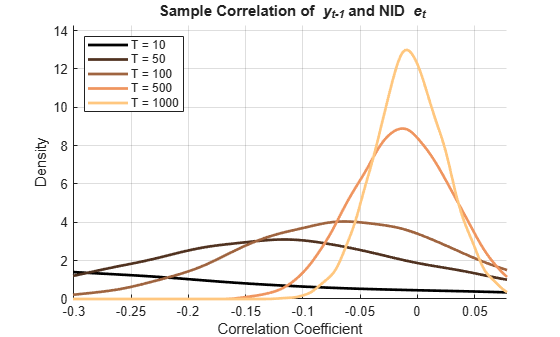figure(hFigLE1) xlabel('Correlation Coefficient') ylabel('Density') title('{\bf Sample Correlation of {\it y_{t-1}} and NID {\it e_{t-1}}}') legend(hPlotsLE1,strcat({'T = '},num2str(T','%-d')),'Location','NW') axis tight grid on ylim([0 (1.1)*max(fLE1)]) hold off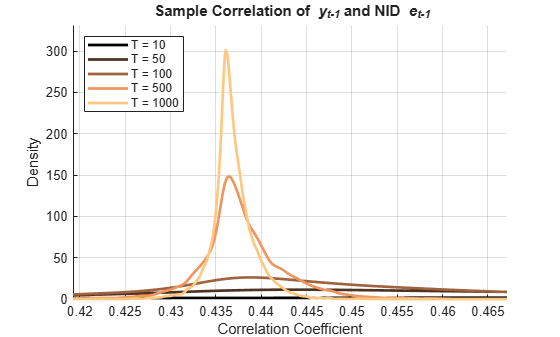figure(hFigE2) xlabel('Correlation Coefficient') ylabel('Density') title('{\bf Sample Correlation of {\it y_{t-1}} and AR(1) {\it e_t}}') legend(hPlotsE2,strcat({'T = '},num2str(T','%-d')),'Location','NW') axis tight grid on ylim([0 (1.1)*max(fE2)]) hold off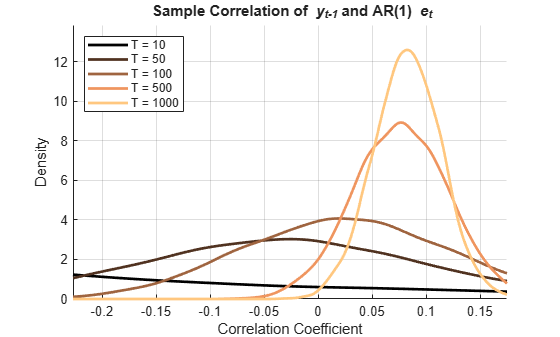figure(hFigLE2) xlabel('Correlation Coefficient') ylabel('Density') title('{\bf Sample Correlation of {\it y_{t-1}} and AR(1) {\it e_{t-1}}}') legend(hPlotsLE2,strcat({'T = '},num2str(T','%-d')),'Location','NW') axis tight grid on ylim([0 (1.1)*max(fLE2)]) hold off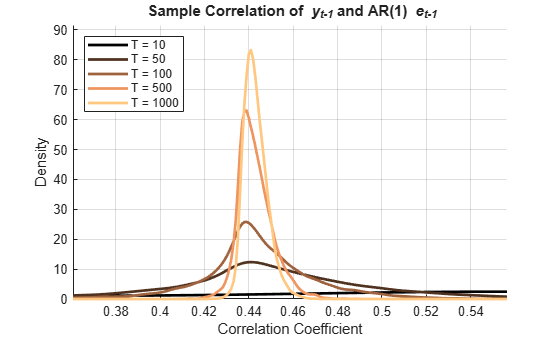The plots show correlation between ${y}_{t-1}$ and ${e}_{t-1}$ in both cases. Contemporaneous correlation between ${y}_{t-1}$ and ${e}_{t}$, however, persists asymptotically only in the case of AR(1) innovations.

The correlation coefficient is the basis for standard measures of autocorrelation. The plots above highlight the bias and variance of the correlation coefficient in finite samples, which complicates the practical evaluation of autocorrelations in model residuals. Correlation measures were examined extensively by Fisher (,,), who suggested a number of alternatives.

Using biased estimates of ${\beta }_{0}$ to estimate ${\gamma }_{0}$ in the residuals is also biased . As described earlier, OLS residuals in the case of AR(1) innovations do not accurately represent process innovations, because of the tendency for $\underset{}{\overset{ˆ}{{\beta }_{0}}}$ to absorb the systematic impact produced by autocorrelated disturbances.

To further complicate matters, the Durbin-Watson statistic, popularly reported as a measure of the degree of first-order autocorrelation, is biased against detecting any relationship between $\underset{}{\overset{ˆ}{{e}_{t}}}$ and ${\underset{}{\overset{ˆ}{e}}}_{t-1}$ in exactly the AR models where such a relationship is present. The bias is twice as large as the bias in $\underset{}{\overset{ˆ}{{\beta }_{0}}}$ .

Thus, OLS can persistently overestimate ${\beta }_{0}$ while standard measures of residual autocorrelation underestimate the conditions that lead to inconsistency. This produces a distorted sense of goodness of fit, and a misrepresentation of the significance of dynamic terms. Durbin's $h$ test is similarly ineffective in this context . Durbin's $m$ test, or the equivalent Breusch-Godfrey test, are often preferred .

### Approximating Estimator Bias

In practice, the process that produces a time series must be discovered from the available data, and this analysis is ultimately limited by the loss of confidence that comes with estimator bias and variance. Sample sizes for economic data are often at the lower end of those considered in the simulations above, so inaccuracies can be significant. Effects on the forecast performance of autoregressive models can be severe.

For simple AR models with simple innovations structures, approximations of the OLS estimator bias are obtained theoretically. These formulas are useful when assessing the reliability of AR model coefficients derived from a single data sample.

In the case of NID innovations, we can compare the simulation bias with the widely-used approximate value of ,:

$E\left[\underset{}{\overset{ˆ}{{\beta }_{0}}}\right]-{\beta }_{0}\approx -2{\beta }_{0}/T.$

m = min(T); M = max(T); eBias1 = AggBetaHat1-beta0; % Estimated bias tBias1 = -2*beta0./T; % Theoretical bias eB1interp = interp1(T,eBias1,m:M,'pchip'); tB1interp = interp1(T,tBias1,m:M,'pchip'); figure plot(T,eBias1,'ro','LineWidth',2) hold on he1 = plot(m:M,eB1interp,'r','LineWidth',2); plot(T,tBias1,'bo') ht1 = plot(m:M,tB1interp,'b'); hold off legend([he1 ht1],'Simulated Bias','Approximate Theoretical Bias','Location','E') xlabel('Sample Size') ylabel('Bias') title('{\bf Estimator Bias, NID Innovations}') grid on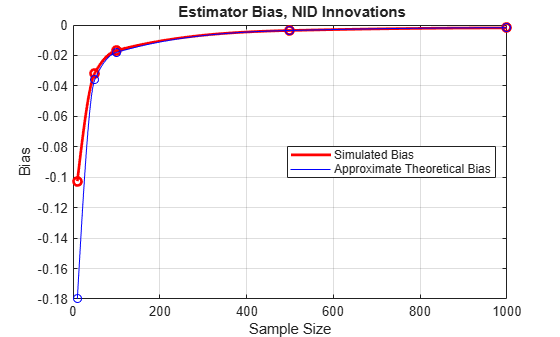The approximation is reasonably reliable in even moderately-sized samples, and generally improves as ${\beta }_{0}$ decreases in absolute value.

In the case of AR(1) innovations, the bias depends on both ${\beta }_{0}$ and ${\gamma }_{0}$. Asymptotically, it is approximated by :

$E\left[\underset{}{\overset{ˆ}{{\beta }_{0}}}\right]-{\beta }_{0}\approx {\gamma }_{0}\left(1-{\beta }_{0}^{2}\right)/\left(1+{\gamma }_{0}{\beta }_{0}\right).$

eBias2 = AggBetaHat2-beta0; % Estimated bias tBias2 = gamma0*(1-beta0^2)/(1+gamma0*beta0); % Asymptotic bias eB2interp = interp1(T,eBias2,m:M,'pchip'); figure plot(T,eBias2,'ro','LineWidth',2) hold on he2 = plot(m:M,eB2interp,'r','LineWidth',2); ht2 = plot(0:M,repmat(tBias2,1,M+1),'b','LineWidth',2); hold off legend([he2 ht2],'Simulated Bias','Approximate Asymptotic Bias','Location','E') xlabel('Sample Size') ylabel('Bias') title('{\bf Estimator Bias, AR(1) Innovations}') grid onHere we see the bias move from negative to positive values as the sample size increases, then eventually approach the asymptotic bound. There is a range of sample sizes, from about 25 to 100, where the absolute value of the bias is below 0.02. In such a "sweet spot," the OLS estimator may outperform alternative estimators designed to specifically account for the presence of autocorrelation. We describe this behavior further in the section Dynamic and Correlation Effects.

It is useful to plot the approximate asymptotic bias in $\underset{}{\overset{ˆ}{{\beta }_{0}}}$ as a function of both ${\beta }_{0}$ and ${\gamma }_{0}$, to see the affect of varying the degree of autocorrelation in both ${y}_{t}$ and ${e}_{t}$:

figure beta = -1:0.05:1; gamma = -1:0.05:1; [Beta,Gamma] = meshgrid(beta,gamma); hold on surf(Beta,Gamma,Gamma.*(1-Beta.^2)./(1+Gamma.*Beta)) fig = gcf; CM = fig.Colormap; numC = size(CM,1); zL = zlim; zScale = zL(2)-zL(1); iSim = (tBias2-zL(1))*numC/zScale; cSim = interp1(1:numC,CM,iSim); hSim = plot3(beta0,gamma0,tBias2,'ko','MarkerSize',8,'MarkerFaceColor',cSim); view(-20,20) ax = gca; u = ax.XTick; v = ax.YTick; mesh(u,v,zeros(length(v),length(u)),'FaceAlpha',0.7,'EdgeColor','k','LineStyle',':') hold off legend(hSim,'Simulated Model','Location','Best') xlabel('\beta_0') ylabel('\gamma_0') zlabel('Bias') title('{\bf Approximate Asymptotic Bias}') camlight colorbar grid onThe asymptotic bias becomes significant when ${\beta }_{0}$ and ${\gamma }_{0}$ move in opposite directions away from zero autocorrelation. Of course, the bias may be considerably less in finite samples.

### Dynamic and Correlation Effects

As discussed, the challenges of using OLS for dynamic model estimation arise from violations of CLM assumptions. Two violations are critical, and we discuss their effects here in more detail.

The first is the dynamic effect, caused by the correlation between the predictor ${y}_{t-1}$ and all of the previous innovation ${e}_{t-k}$. This occurs in any AR model, and results in biased OLS estimates from finite samples. In the absence of other violations, OLS nevertheless remains consistent, and the bias disappears in large samples.

The second is the correlation effect, caused by the contemporaneous correlation between the predictor ${y}_{t-1}$ and the innovation ${e}_{t}$. This occurs when the innovations process is autocorrelated, and results in the OLS coefficient of the predictor receiving too much, or too little, credit for contemporaneous variations in the response, depending on the sign of the correlation. That is, it produces a persistent bias.

The first set of simulations above illustrate a situation in which ${\beta }_{0}$ is positive and ${\gamma }_{0}$ is zero. The second set of simulations illustrate a situation in which both ${\beta }_{0}$ and ${\gamma }_{0}$ are positive. For positive ${\beta }_{0}$, the dynamic effect on $\underset{}{\overset{ˆ}{{\beta }_{0}}}$ is negative. For positive ${\gamma }_{0}$, the correlation effect on $\underset{}{\overset{ˆ}{{\beta }_{0}}}$ is positive. Thus, in the first set of simulations there is a negative bias across sample sizes. In the second set of simulations, however, there is a competition between the two effects, with the dynamic effect dominating in small samples, and the correlation effect dominating in large samples.

Positive AR coefficients are common in econometric models, so it is typical for the two effects to offset each other, creating a range of sample sizes for which the OLS bias is significantly reduced. The width of this range depends on ${\beta }_{0}$ and ${\gamma }_{0}$, and determines the OLS-superior range in which OLS outperforms alternative estimators designed to directly account for autocorrelations in the innovations.

Some of the factors affecting the size of the dynamic and correlation effects are summarized in . Among them:

Dynamic Effect

• Increases with decreasing sample size.

• Decreases with increasing ${\beta }_{0}$ if the variance of the innovations is held fixed.

• Decreases with increasing ${\beta }_{0}$ if the variance of the innovations is adjusted to maintain a constant ${R}^{2}$.

• Increases with the variance of the innovations.

Correlation Effect

• Increases with increasing ${\gamma }_{0}$, at a decreasing rate.

• Decreases with increasing ${\beta }_{0}$, at an increasing rate.

The influence of these factors can be tested by changing the coefficients in the simulations above. In general, the larger the dynamic effect and the smaller the correlation effect, the wider the OLS-superior range.

### Jackknife Bias Reduction

The jackknife procedure is a cross-validation technique commonly used to reduce the bias of sample statistics. Jacknife estimators of model coefficients are relatively easy to compute, without the need for large simulations or resampling.

The basic idea is to compute an estimate from the full sample and from a sequence of subsamples, then combine the estimates in a manner that eliminates some portion of the bias. In general, for a sample of size $T$, the bias of the OLS estimator $\underset{}{\overset{ˆ}{{\beta }_{0}}}$ can be expressed as an expansion in powers of ${T}^{-1}$:

$E\left(\underset{}{\overset{ˆ}{{\beta }_{0}}}\right)-{\beta }_{0}=\frac{{w}_{1}}{T}+\frac{{w}_{2}}{{T}^{2}}+O\left({T}^{-3}\right),$

where the weights ${w}_{1}$ and ${w}_{2}$ depend on the specific coefficient and model. If estimates $\underset{}{\overset{ˆ}{{\beta }_{i}}}$ are made on a sequence $i=1,...,m$ of subsamples of length $l=O\left(T\right)$, then the jackknife estimator of ${\beta }_{0}$ is:

$\underset{}{\overset{ˆ}{{\beta }_{J}}}=\left(\frac{T}{T-l}\right)\underset{}{\overset{ˆ}{{\beta }_{0}}}-\left(\frac{l}{T-l}\right)\frac{1}{m}\sum _{i=1}^{m}\underset{}{\overset{ˆ}{{\beta }_{i}}}.$

It can be shown that the jackknife estimator satisfies:

$E\left(\underset{}{\overset{ˆ}{{\beta }_{0}}}\right)-{\beta }_{0}=O\left({T}^{-2}\right),$

thus removing the $O\left({T}^{-1}\right)$ term from the bias expansion. Whether the bias is actually reduced depends on the size of the remaining terms in the expansion, but jackknife estimators have performed well in practice. In particular, the technique is robust with respect to nonnormal innovations, ARCH effects, and various model misspecifications .

The Statistics and Machine Learning Toolbox™ function jackknife implements a jackknife procedure using a systematic sequence of "leave-one-out" subsamples. For time series, deleting observations alters the autocorrelation structure. To maintain the dependence structure in a time series, a jackknife procedure must use nonoverlapping subsamples, such as partitions or moving blocks.

The following implements a simple jackknife estimate of $\underset{}{\overset{ˆ}{{\beta }_{0}}}$ using a partition of the data in each of the simulations to produce the subsample estimates $\underset{}{\overset{ˆ}{{\beta }_{i}}}$. We compare the performance before and after jackknifing, on the simulated data with either NID or AR(1) innovations:

m = 5; % Number of subsamples % Preallocate memory: betaHat1 = zeros(m,1); % Subsample estimates, NID innovations betaHat2 = zeros(m,1); % Subsample estimates, AR(1) innovations BetaHat1J = zeros(numSizes,numPaths); % Jackknife estimates, NID innovations BetaHat2J = zeros(numSizes,numPaths); % Jackknife estimates, AR(1) innovations % Compute jackknife estimates: for i = 1:numSizes n = T(i); % Sample size l = n/m; % Length of partition subinterval for j = 1:numPaths for s = 1:m betaHat1(s) = LY1((s-1)*l+1:s*l,j)\Y1((s-1)*l+1:s*l,j); betaHat2(s) = LY2((s-1)*l+1:s*l,j)\Y2((s-1)*l+1:s*l,j); BetaHat1J(i,j) = (n/(n-l))*BetaHat1(i,j)-(l/((n-l)*m))*sum(betaHat1); BetaHat2J(i,j) = (n/(n-l))*BetaHat2(i,j)-(l/((n-l)*m))*sum(betaHat2); end end end clear BetaHat1 BetaHat2 % Display mean estimates, before and after jackknifing: AggBetaHat1J = mean(BetaHat1J,2); clear BetaHat1J fprintf('%-6s%-8s%-8s\n','Size','Mean1','Mean1J')
Size Mean1 Mean1J 
for i = 1:numSizes fprintf('%-6u%-8.4f%-8.4f\n',T(i),AggBetaHat1(i),AggBetaHat1J(i)) end
10 0.7974 0.8055 50 0.8683 0.8860 100 0.8833 0.8955 500 0.8964 0.8997 1000 0.8981 0.8998 
AggBetaHat2J = mean(BetaHat2J,2); clear BetaHat2J fprintf('%-6s%-8s%-8s\n','Size','Mean2','Mean2J')
Size Mean2 Mean2J 
for i = 1:numSizes fprintf('%-6u%-8.4f%-8.4f\n',T(i),AggBetaHat2(i),AggBetaHat2J(i)) end
10 0.8545 0.8594 50 0.9094 0.9233 100 0.9201 0.9294 500 0.9299 0.9323 1000 0.9310 0.9323 

The number of subsamples, $m=5$, is chosen with the smallest sample size, $n=10$, in mind. Larger $m$ may improve performance in larger samples, but there is no accepted heuristic for choosing the subsample sizes, so some experimentation is necessary. The code is easily adapted to use alternative subsampling methods, such as moving blocks.

The results show a uniform reduction in bias for the case of NID innovations. In the case of AR(1) innovations, the procedure seems to push the estimate more quickly through the OLS-superior range.

### Summary

This example shows a simple AR model, together with a few simple innovations structures, as a way of illustrating some general issues related to the estimation of dynamic models. The code here is easily modified to observe the effects of changing parameter values, adjusting the innovations variance, using different lag structures, and so on. Explanatory DL terms can also be added to the models. DL terms have the ability to reduce estimator bias, though OLS tends to overestimate AR coefficients at the expense of the DL coefficients . The general set-up here allows for a great deal of experimentation, as is often required when evaluating models in practice.

When considering the trade-offs presented by the bias and variance of any estimator, it is important to remember that biased estimators with reduced variance may have superior mean-squared error characteristics when compared to higher-variance unbiased estimators. A strong point of the OLS estimator, beyond its simplicity in computation, is its relative efficiency in reducing its variance with increasing sample size. This is often enough to adopt OLS as the estimator of choice, even for dynamic models. Another strong point, as this example has shown, is the presence of an OLS-superior range, where OLS may outperform other estimators, even under what are generally regarded as adverse conditions. The weakest point of the OLS estimator is its performance in small samples, where the bias and variance may be unacceptable.

The estimation issues raised in this example suggest the need for new indicators of autocorrelation, and more robust estimation methods to be used in its presence. Some of these methods are described in the example Time Series Regression X: Generalized Least Squares and HAC Estimators. However, as we have seen, the inconsistency of the OLS estimator for AR models with autocorrelation is not enough to rule it out, in general, as a viable competitor to more complicated, consistent estimators such as maximum likelihood, feasible generalized least squares, and instrumental variables, which attempt to eliminate the correlation effect, but do not alter the dynamic effect. The best choice will depend on the sample size, the lag structure, the presence of exogenous variables, and so on, and often requires the kinds of simulations presented in this example.

 Breusch, T.S., and L. G. Godfrey. "A Review of Recent Work on Testing for Autocorrelation in Dynamic Simultaneous Models." In Currie, D., R. Nobay, and D. Peel (Eds.), Macroeconomic Analysis: Essays in Macroeconomics and Econometrics. London: Croom Helm, 1981.

 Chambers, M. J. "Jackknife Estimation of Stationary Autoregressive Models." University of Essex Discussion Paper No. 684, 2011.

 Fisher, R. A.. "Frequency Distribution of the Values of the Correlation Coefficient in Samples from an Indefinitely Large Population." Biometrika. Vol. 10, 1915, pp. 507–521.

 Fisher, R. A. "On the "Probable Error" of a Coefficient of Correlation Deduced from a Small Sample." Metron. Vol. 1, 1921, pp. 3–32.

 Fisher, R. A. "The Distribution of the Partial Correlation Coefficient." Metron. Vol. 3, 1924, pp. 329–332.

 Hibbs, D. "Problems of Statistical Estimation and Causal Inference in Dynamic Time Series Models." In Costner, H. (Ed.) Sociological Methodology. San Francisco: Jossey-Bass, 1974.

 Inder, B. A. "Finite Sample Power of Tests for Autocorrelation in Models Containing Lagged Dependent Variables." Economics Letters. Vol. 14, 1984, pp.179–185.

 Johnston, J. Econometric Methods. New York: McGraw-Hill, 1972.

 Maeshiro, A. "Teaching Regressions with a Lagged Dependent Variable and Autocorrelated Disturbances." Journal of Economic Education. Vol. 27, 1996, pp. 72–84.

 Maeshiro, A. "An Illustration of the Bias of OLS for Yt = λYt–1+Ut." Journal of Economic Education. Vol. 31, 2000, pp. 76–80.

 Malinvaud, E. Statistical Methods of Econometrics. Amsterdam: North-Holland, 1970.

 Marriott, F. and J. Pope. "Bias in the Estimation of Autocorrelations." Biometrika. Vol. 41, 1954, pp. 390–402.

 White, J. S. "Asymptotic Expansions for the Mean and Variance of the Serial Correlation Coefficient." Biometrika. Vol 48, 1961, pp. 85–94.